# Support Vector Machine (SVM)

## Introduction to SVM

Support vector machines (SVMs) are powerful yet flexible supervised machine learning algorithms which are used both for classification and regression. But generally, they are used in classification problems. In 1960s, SVMs were first introduced but later they got refined in 1990. SVMs have their unique way of implementation as compared to other machine learning algorithms. Lately, they are extremely popular because of their ability to handle multiple continuous and categorical variables.

## Working of SVM

An SVM model is basically a representation of different classes in a hyperplane in multidimensional space. The hyperplane will be generated in an iterative manner by SVM so that the error can be minimized. The goal of SVM is to divide the datasets into classes to find a maximum marginal hyperplane (MMH).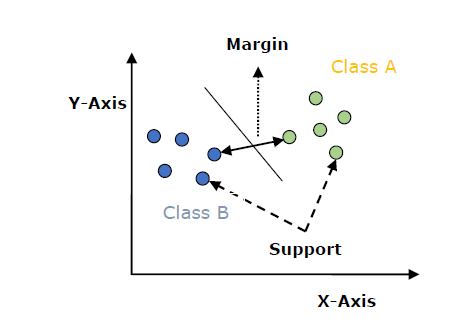The followings are important concepts in SVM −

• Support Vectors − Datapoints that are closest to the hyperplane is called support vectors. Separating line will be defined with the help of these data points.

• Hyperplane − As we can see in the above diagram, it is a decision plane or space which is divided between a set of objects having different classes.

• Margin − It may be defined as the gap between two lines on the closet data points of different classes. It can be calculated as the perpendicular distance from the line to the support vectors. Large margin is considered as a good margin and small margin is considered as a bad margin.

The main goal of SVM is to divide the datasets into classes to find a maximum marginal hyperplane (MMH) and it can be done in the following two steps −

• First, SVM will generate hyperplanes iteratively that segregates the classes in best way.

• Then, it will choose the hyperplane that separates the classes correctly.

## Implementing SVM in Python

For implementing SVM in Python we will start with the standard libraries import as follows −

import numpy as np
import matplotlib.pyplot as plt
from scipy import stats
import seaborn as sns; sns.set()


Next, we are creating a sample dataset, having linearly separable data, from sklearn.dataset.sample_generator for classification using SVM −

from sklearn.datasets.samples_generator import make_blobs
X, y = make_blobs(n_samples=100, centers=2, random_state=0, cluster_std=0.50)
plt.scatter(X[:, 0], X[:, 1], c=y, s=50, cmap='summer');


The following would be the output after generating sample dataset having 100 samples and 2 clusters −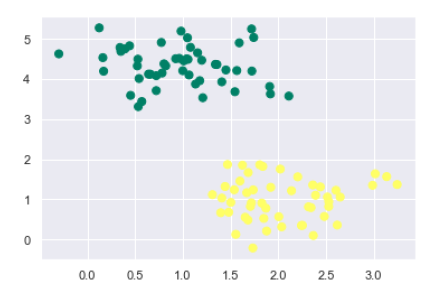We know that SVM supports discriminative classification. it divides the classes from each other by simply finding a line in case of two dimensions or manifold in case of multiple dimensions. It is implemented on the above dataset as follows −

xfit = np.linspace(-1, 3.5)
plt.scatter(X[:, 0], X[:, 1], c=y, s=50, cmap='summer')
plt.plot([0.6], [2.1], 'x', color='black', markeredgewidth=4, markersize=12)
for m, b in [(1, 0.65), (0.5, 1.6), (-0.2, 2.9)]:
plt.plot(xfit, m * xfit + b, '-k')
plt.xlim(-1, 3.5);


The output is as follows −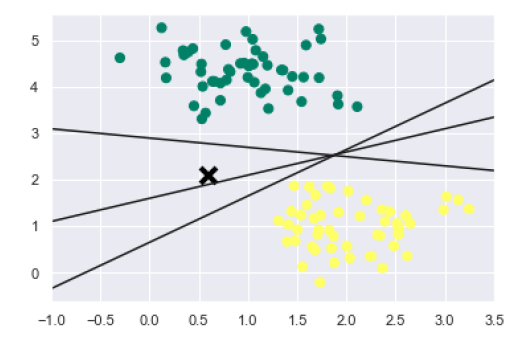We can see from the above output that there are three different separators that perfectly discriminate the above samples.

As discussed, the main goal of SVM is to divide the datasets into classes to find a maximum marginal hyperplane (MMH) hence rather than drawing a zero line between classes we can draw around each line a margin of some width up to the nearest point. It can be done as follows −

xfit = np.linspace(-1, 3.5)
plt.scatter(X[:, 0], X[:, 1], c=y, s=50, cmap='summer')
for m, b, d in [(1, 0.65, 0.33), (0.5, 1.6, 0.55), (-0.2, 2.9, 0.2)]:
yfit = m * xfit + b
plt.plot(xfit, yfit, '-k')
plt.fill_between(xfit, yfit - d, yfit + d, edgecolor='none',
color='#AAAAAA', alpha=0.4)
plt.xlim(-1, 3.5);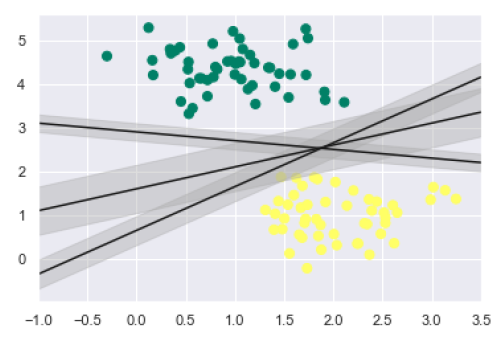From the above image in output, we can easily observe the “margins” within the discriminative classifiers. SVM will choose the line that maximizes the margin.

Next, we will use Scikit-Learn’s support vector classifier to train an SVM model on this data. Here, we are using linear kernel to fit SVM as follows −

from sklearn.svm import SVC # "Support vector classifier"
model = SVC(kernel='linear', C=1E10)
model.fit(X, y)


The output is as follows −

SVC(C=10000000000.0, cache_size=200, class_weight=None, coef0=0.0,
decision_function_shape='ovr', degree=3, gamma='auto_deprecated',
kernel='linear', max_iter=-1, probability=False, random_state=None,
shrinking=True, tol=0.001, verbose=False)


Now, for a better understanding, the following will plot the decision functions for 2D SVC −

def decision_function(model, ax=None, plot_support=True):
if ax is None:
ax = plt.gca()
xlim = ax.get_xlim()
ylim = ax.get_ylim()


For evaluating model, we need to create grid as follows −

x = np.linspace(xlim, xlim, 30)
y = np.linspace(ylim, ylim, 30)
Y, X = np.meshgrid(y, x)
xy = np.vstack([X.ravel(), Y.ravel()]).T
P = model.decision_function(xy).reshape(X.shape)


Next, we need to plot decision boundaries and margins as follows −

ax.contour(X, Y, P, colors='k',
levels=[-1, 0, 1], alpha=0.5,
linestyles=['--', '-', '--'])


Now, similarly plot the support vectors as follows −

if plot_support:
ax.scatter(model.support_vectors_[:, 0],
model.support_vectors_[:, 1],
s=300, linewidth=1, facecolors='none');
ax.set_xlim(xlim)
ax.set_ylim(ylim)


Now, use this function to fit our models as follows −

plt.scatter(X[:, 0], X[:, 1], c=y, s=50, cmap='summer')
decision_function(model);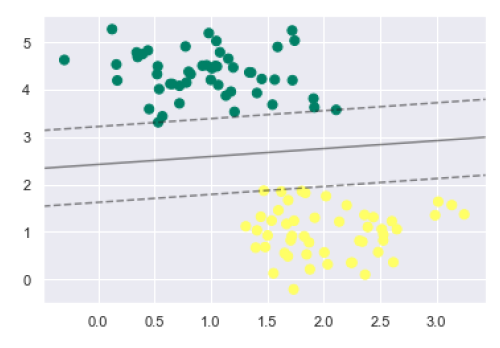We can observe from the above output that an SVM classifier fit to the data with margins i.e. dashed lines and support vectors, the pivotal elements of this fit, touching the dashed line. These support vector points are stored in the support_vectors_ attribute of the classifier as follows −

model.support_vectors_


The output is as follows −

array([[0.5323772 , 3.31338909],
[2.11114739, 3.57660449],
[1.46870582, 1.86947425]])


## SVM Kernels

In practice, SVM algorithm is implemented with kernel that transforms an input data space into the required form. SVM uses a technique called the kernel trick in which kernel takes a low dimensional input space and transforms it into a higher dimensional space. In simple words, kernel converts non-separable problems into separable problems by adding more dimensions to it. It makes SVM more powerful, flexible and accurate. The following are some of the types of kernels used by SVM −

### Linear Kernel

It can be used as a dot product between any two observations. The formula of linear kernel is as below −

k(x,xi) = sum(x*xi)

From the above formula, we can see that the product between two vectors say 𝑥 & 𝑥𝑖 is the sum of the multiplication of each pair of input values.

### Polynomial Kernel

It is more generalized form of linear kernel and distinguish curved or nonlinear input space. Following is the formula for polynomial kernel −

K(x, xi) = 1 + sum(x * xi)^d

Here d is the degree of polynomial, which we need to specify manually in the learning algorithm.

### Radial Basis Function (RBF) Kernel

RBF kernel, mostly used in SVM classification, maps input space in indefinite dimensional space. Following formula explains it mathematically −

K(x,xi) = exp(-gamma * sum((x – xi^2))

Here, gamma ranges from 0 to 1. We need to manually specify it in the learning algorithm. A good default value of gamma is 0.1.

As we implemented SVM for linearly separable data, we can implement it in Python for the data that is not linearly separable. It can be done by using kernels.

### Example

The following is an example for creating an SVM classifier by using kernels. We will be using iris dataset from scikit-learn −

We will start by importing following packages −

import pandas as pd
import numpy as np
from sklearn import svm, datasets
import matplotlib.pyplot as plt


Now, we need to load the input data −

iris = datasets.load_iris()


From this dataset, we are taking first two features as follows −

X = iris.data[:, :2]
y = iris.target


Next, we will plot the SVM boundaries with original data as follows −

x_min, x_max = X[:, 0].min() - 1, X[:, 0].max() + 1
y_min, y_max = X[:, 1].min() - 1, X[:, 1].max() + 1
h = (x_max / x_min)/100
xx, yy = np.meshgrid(np.arange(x_min, x_max, h),
np.arange(y_min, y_max, h))
X_plot = np.c_[xx.ravel(), yy.ravel()]


Now, we need to provide the value of regularization parameter as follows −

C = 1.0


Next, SVM classifier object can be created as follows −

Svc_classifier = svm.SVC(kernel='linear', C=C).fit(X, y)

Z = svc_classifier.predict(X_plot)
Z = Z.reshape(xx.shape)
plt.figure(figsize=(15, 5))
plt.subplot(121)
plt.contourf(xx, yy, Z, cmap=plt.cm.tab10, alpha=0.3)
plt.scatter(X[:, 0], X[:, 1], c=y, cmap=plt.cm.Set1)
plt.xlabel('Sepal length')
plt.ylabel('Sepal width')
plt.xlim(xx.min(), xx.max())
plt.title('Support Vector Classifier with linear kernel')


### Output

Text(0.5, 1.0, 'Support Vector Classifier with linear kernel')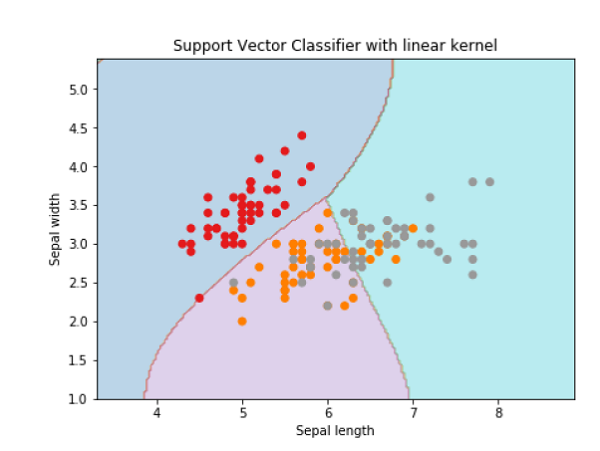For creating SVM classifier with rbf kernel, we can change the kernel to rbf as follows −

Svc_classifier = svm.SVC(kernel='rbf', gamma =‘auto’,C=C).fit(X, y)
Z = svc_classifier.predict(X_plot)
Z = Z.reshape(xx.shape)
plt.figure(figsize=(15, 5))
plt.subplot(121)
plt.contourf(xx, yy, Z, cmap=plt.cm.tab10, alpha=0.3)
plt.scatter(X[:, 0], X[:, 1], c=y, cmap=plt.cm.Set1)
plt.xlabel('Sepal length')
plt.ylabel('Sepal width')
plt.xlim(xx.min(), xx.max())
plt.title('Support Vector Classifier with rbf kernel')


### Output

Text(0.5, 1.0, 'Support Vector Classifier with rbf kernel')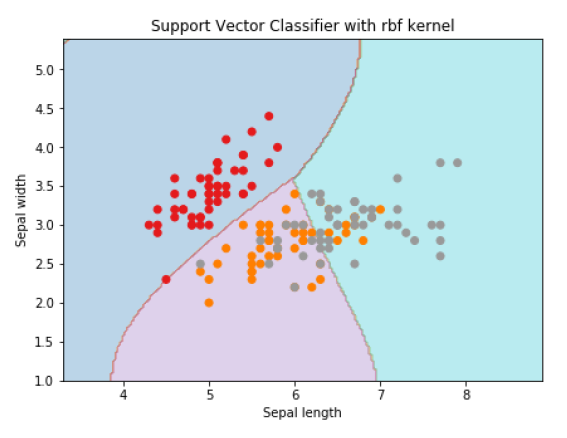We put the value of gamma to ‘auto’ but you can provide its value between 0 to 1 also.

## Pros and Cons of SVM Classifiers

### Pros of SVM classifiers

SVM classifiers offers great accuracy and work well with high dimensional space. SVM classifiers basically use a subset of training points hence in result uses very less memory.

### Cons of SVM classifiers

They have high training time hence in practice not suitable for large datasets. Another disadvantage is that SVM classifiers do not work well with overlapping classes.